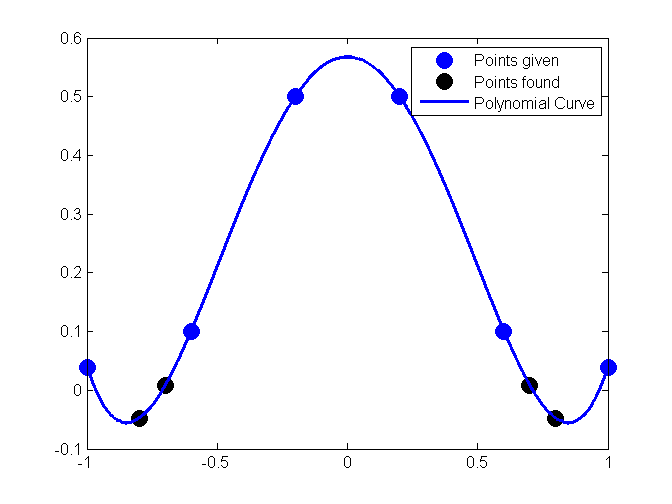# HOW DO I DO THAT IN MATLAB SERIES?

In this series, I am answering questions that students have asked me about MATLAB. Most of the questions relate to a mathematical procedure.

## TOPIC

How do I do polynomial interpolation?

## SUMMARY

```% Language : Matlab 2008a;
% Authors : Autar Kaw;
% Mfile available at
% http://numericalmethods.eng.usf.edu/blog/interpolate_polynomial.m;
% Last Revised : June 10, 2009;
% Abstract: This program shows you how to do polynomial interpolation?
%           .
clc
clear all
clf
```

## INTRODUCTION

```disp('ABSTRACT')
disp('   This program shows you how to do polynomial interpolation?')
disp(' ')
disp('AUTHOR')
disp('   Autar K Kaw of http://autarkaw.wordpress.com')
disp(' ')
disp('MFILE SOURCE')
disp('   http://numericalmethods.eng.usf.edu/blog/interpolation_polynomial.m')
disp(' ')
disp('LAST REVISED')
disp('   June 10, 2009')
disp(' ')
```
```ABSTRACT
This program shows you how to do polynomial interpolation?

AUTHOR
Autar K Kaw of http://autarkaw.wordpress.com

MFILE SOURCE
http://numericalmethods.eng.usf.edu/blog/interpolation_polynomial.m

LAST REVISED
June 10, 2009

```

## INPUTS

y vs x data to interpolate x data

```x=[-1  -0.6  -0.2  0.2  0.6  1];
% ydata
y=[0.0385    0.1000    0.5000    0.5000    0.1000    0.0385];
% Where do you want to interpolate at
xin=[-0.8  -0.7  0.7  0.8];
```

## DISPLAYING INPUTS

```disp('INPUTS')
disp('The x data')
x
disp('The y data')
y
disp('The x values where you want to find the interpolated values')
xin
disp('  ')
```
```INPUTS
The x data

x =

-1.0000   -0.6000   -0.2000    0.2000    0.6000    1.0000

The y data

y =

0.0385    0.1000    0.5000    0.5000    0.1000    0.0385

The x values where you want to find the interpolated values

xin =

-0.8000   -0.7000    0.7000    0.8000

```

## THE CODE

Find the number of data points

```n=length(x);
% Fitting to polynomial of order m=n-1
m=n-1
% pp consists of the coefficients of the polynomial
% pp(1)*x^m+pp(2)*x^m+.......+pp(m)
% pp(1) is coefficient of x^m
% pp(2) is coefficient of x^(m-1)
% and so on
pp=polyfit(x,y,m);
% Getting the values at xin
yin=polyval(pp,xin);
% This is only for plotting the interpolating polynomial
xplot=x(1):(x(n)-x(1))/10000:x(n);
yplot=polyval(pp,xplot);
```
```m =

5

```

## DISPLAYING OUTPUTS

```disp('  ')
disp('OUTPUTS')
disp('x values at which function is to be interpolated')
xin
disp('y values at the xin values')
yin
disp('These are the coefficients of the polynomial interpolant')
disp('pp(1) is coefficient of x^m, pp(2) is coefficient of x^(m-1) and so on')
fprintf('Order of polynomial m =%g',m)
pp
xlabel('x');
ylabel('y');
title('y vs x ');
plot(x,y,'o','MarkerSize',10,'MarkerEdgeColor','b','MarkerFaceColor','b')
hold on
plot(xin,yin,'o','MarkerSize',10,'MarkerEdgeColor','k','MarkerFaceColor','k')
hold on
plot(xplot,yplot,'LineWidth',2)
legend('Points given','Points found','Polynomial Curve')
hold off
disp('  ')
```
```
OUTPUTS
x values at which function is to be interpolated

xin =

-0.8000   -0.7000    0.7000    0.8000

y values at the xin values

yin =

-0.0481    0.0078    0.0078   -0.0481

These are the coefficients of the polynomial interpolant
pp(1) is coefficient of x^m, pp(2) is coefficient of x^(m-1) and so on
Order of polynomial m =5
pp =

0.0000    1.2020   -0.0000   -1.7308    0.0000    0.5673

```